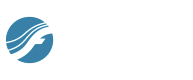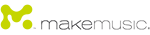You are here: How do I... > Advanced configurations > Customized Speedy Keymap

# Customized Speedy Entry keymap

This section describes how to install a customized Speedy Entry keymap for German and Swiss-German keyboards for Finale for Windows. The techniques can also be used for other international keyboards. Installing the customized keymap requires editing your FINALE.INI file directly. Use caution when editing FINALE.INI: follow the warnings and instructions below.

The FINALE.INI file can be edited with a text editor such as Notepad. If you use a word processor (such as Microsoft Word), be sure to save the file as “text only with line breaks”. Do not edit the FINALE.INI file while Finale is running.

WARNING! If you choose to edit the FINALE.INI file, be sure to make a backup of it first. This is the ONLY recourse for correcting any mistakes. If you make an accidental change, you can either restore the backup copy of the FINALE.INI file, or delete the edited FINALE.INI file. When you launch Finale again, Finale re-generates a new FINALE.INI. If Finale creates a new FINALE.INI file, any custom settings and Finale Preferences you had saved in your previous INI file will be lost.

If you have any doubts about how to edit FINALE.INI, don't do it! Mistakes can cause you to lose your Finale settings, and perhaps cause Finale to run improperly. This is why a backup copy of the file is so important.

Noteman says: When you install a new version of Finale on your computer, customized keymaps are not copied from the previous version. To migrate these settings, you must follow these instructions again with the new FINALE.INI file.

The FINALE.INI file is divided into sections, which are separated by headers that appear in square brackets. You will need to replace the entire [SpeedyKeys] section of FINALE.INI, including the line “[SpeedyKeys]” as well as all subsequent lines beginning with “SK”. Replace the [SpeedyKeys] section with the new [SpeedyKeys] section below. If the [SpeedyKeys] section does not exist in your FINALE.INI, simply add the new section below.

This keymap adopts the standard Speedy Entry commands for German and Swiss-German keyboard layouts. In addition, Swiss-German users can now use the '\$' key to change layers (instead of the '#' key).

[SpeedyKeys]

SK000=59 0 -1 -1 -1 -1 5

SK001=65549 0 0 -1 -1 -1 34

SK002=65582 0 -1 -1 -1 -1 23

SK003=65544 0 0 -1 -1 -1 24

SK004=65574 0 0 -1 -1 -1 15

SK005=65574 0 1 -1 -1 -1 11

SK006=65576 0 0 -1 -1 -1 16

SK007=65576 0 1 -1 -1 -1 12

SK008=65573 0 0 -1 -1 -1 13

SK009=65573 0 1 -1 -1 -1 9

SK010=65573 1 0 -1 -1 -1 17

SK011=65575 0 0 -1 -1 -1 14

SK012=65575 0 1 -1 -1 -1 10

SK013=65575 1 0 -1 -1 -1 18

SK014=91 0 -1 -1 -1 -1 9

SK015=93 0 -1 -1 -1 -1 10

SK016=65563 0 0 -1 -1 -1 82

SK017=39 0 -1 -1 -1 -1 6

SK018=35 0 -1 -1 -1 -1 8

SK019=65725 0 1 -1 -1 -1 25

SK020=65725 1 1 -1 -1 -1 26

SK021=65723 0 0 -1 -1 -1 19

SK022=65723 1 0 -1 -1 -1 21

SK023=65725 0 0 -1 -1 -1 20

SK024=65725 1 0 -1 -1 -1 22

SK025=65643 0 -1 -1 -1 -1 19

SK026=65643 1 -1 -1 -1 -1 21

SK027=65645 0 -1 -1 -1 -1 20

SK028=65645 1 -1 -1 -1 -1 22

SK029=65642 0 -1 -1 -1 -1 28

SK030=65642 1 -1 -1 -1 -1 29

SK031=47 0 -1 -1 -1 -1 27

SK032=46 0 -1 -1 -1 -1 33

SK033=65723 0 1 -1 -1 -1 28

SK034=65723 1 1 -1 -1 -1 29

SK035=65615 0 -1 -1 -1 -1 1

SK036=65616 0 -1 -1 -1 -1 2

SK037=65612 0 -1 -1 -1 -1 3

SK038=65612 1 -1 -1 -1 -1 4

SK039=65584 0 0 -1 -1 -1 81

SK040=65584 1 0 -1 -1 -1 39

SK041=65584 0 1 -1 -1 -1 48

SK042=65584 1 1 -1 -1 -1 104

SK043=65585 0 0 -1 -1 -1 40

SK044=65585 0 1 -1 -1 -1 49

SK045=65585 1 1 -1 -1 -1 105

SK046=65585 1 0 -1 -1 -1 83

SK047=65586 0 0 -1 -1 -1 41

SK048=65586 0 1 -1 -1 -1 50

SK049=65586 1 1 -1 -1 -1 106

SK050=65586 1 0 -1 -1 -1 84

SK051=65587 0 0 -1 -1 -1 42

SK052=65587 0 1 -1 -1 -1 51

SK053=65587 1 1 -1 -1 -1 107

SK054=65587 1 0 -1 -1 -1 85

SK055=65588 0 0 -1 -1 -1 43

SK056=65588 0 1 -1 -1 -1 52

SK057=65588 1 1 -1 -1 -1 108

SK058=65588 1 0 -1 -1 -1 86

SK059=65589 0 0 -1 -1 -1 44

SK060=65589 0 1 -1 -1 -1 53

SK061=65589 1 1 -1 -1 -1 109

SK062=65589 1 0 -1 -1 -1 87

SK063=65590 0 0 -1 -1 -1 45

SK064=65590 0 1 -1 -1 -1 54

SK065=65590 1 1 -1 -1 -1 110

SK066=65590 1 0 -1 -1 -1 88

SK067=65591 0 0 -1 -1 -1 46

SK068=65591 0 1 -1 -1 -1 55

SK069=65591 1 1 -1 -1 -1 111

SK070=65591 1 0 -1 -1 -1 89

SK071=65592 0 0 -1 -1 -1 47

SK072=65592 0 1 -1 -1 -1 56

SK073=65592 1 1 -1 -1 -1 112

SK074=65592 1 0 -1 -1 -1 90

SK075=65593 1 0 -1 -1 -1 32

SK076=65593 0 0 -1 -1 -1 31

SK077=65632 0 -1 -1 -1 -1 81

SK078=65632 1 0 -1 -1 -1 39

SK079=65633 0 -1 -1 -1 -1 40

SK080=65633 1 -1 -1 -1 -1 83

SK081=65634 0 -1 -1 -1 -1 41

SK082=65634 1 -1 -1 -1 -1 84

SK083=65635 0 -1 -1 -1 -1 42

SK084=65635 1 -1 -1 -1 -1 85

SK085=65636 0 -1 -1 -1 -1 43

SK086=65636 1 -1 -1 -1 -1 86

SK087=65637 0 -1 -1 -1 -1 44

SK088=65637 1 -1 -1 -1 -1 87

SK089=65638 0 -1 -1 -1 -1 45

SK090=65638 1 -1 -1 -1 -1 88

SK091=65639 0 -1 -1 -1 -1 46

SK092=65639 1 -1 -1 -1 -1 89

SK093=65640 0 -1 -1 -1 -1 47

SK094=65640 1 -1 -1 -1 -1 90

SK095=65641 0 -1 -1 -1 -1 31

SK096=65641 1 -1 -1 -1 -1 32

SK097=65609 0 -1 1 -1 0 57

SK098=65611 0 -1 1 -1 0 58

SK099=65724 0 0 1 -1 0 59

SK100=65625 0 -1 1 -1 0 60

SK101=65624 0 -1 1 -1 0 61

SK102=65603 0 -1 1 -1 0 62

SK103=65622 0 -1 1 -1 0 63

SK104=65602 0 -1 1 -1 0 64

SK105=65614 0 -1 1 -1 0 65

SK106=65613 0 -1 1 -1 0 66

SK107=65601 0 -1 1 -1 0 67

SK108=65619 0 -1 1 -1 0 68

SK109=65604 0 -1 1 -1 0 69

SK110=65606 0 -1 1 -1 0 70

SK111=65607 0 -1 1 -1 0 71

SK112=65608 0 -1 1 -1 0 72

SK113=65610 0 -1 1 -1 0 73

SK114=65617 0 -1 1 -1 0 74

SK115=65623 0 -1 1 -1 0 75

SK116=65605 0 -1 1 -1 0 76

SK117=65618 0 -1 1 -1 0 77

SK118=65620 0 -1 1 -1 0 78

SK119=65626 0 -1 1 -1 0 79

SK120=65621 0 -1 1 -1 0 80

SK121=65646 0 0 -1 -1 -1 33

SK122=65759 0 0 -1 -1 -1 8

SK123=246 -1 -1 -1 -1 -1 100

SK124=214 -1 -1 -1 -1 -1 100

SK125=228 -1 -1 -1 -1 -1 101

SK126=196 -1 -1 -1 -1 -1 101

SK127=65556 0 0 0 -1 -1 102

SK128=65619 0 0 1 -1 1 122

SK129=65619 0 0 0 -1 -1 122

SK130=65619 0 1 1 -1 1 19

SK131=65619 0 1 0 -1 -1 19

SK132=65606 0 0 1 -1 1 123

SK133=65606 0 0 0 -1 -1 123

SK134=65606 0 1 1 -1 1 20

SK135=65606 0 1 0 -1 -1 20

SK136=65614 0 -1 1 -1 1 124

SK137=65614 0 -1 0 -1 -1 124

SK138=65624 0 -1 1 -1 1 125

SK139=65624 0 -1 0 -1 -1 125

SK140=65622 0 -1 1 -1 1 126

SK141=65622 0 -1 0 -1 -1 126

SK142=65602 0 0 1 -1 1 27

SK143=65602 0 0 0 -1 -1 27

SK144=65602 0 1 1 -1 1 128

SK145=65602 0 1 0 -1 -1 128

SK146=65613 0 -1 1 -1 1 100

SK147=65613 0 -1 0 -1 -1 100

SK148=65601 0 -1 1 -1 1 129

SK149=65601 0 -1 0 -1 -1 129

SK150=65607 0 -1 1 -1 1 5

SK151=65607 0 -1 0 -1 -1 5

SK152=65608 0 -1 1 -1 1 1

SK153=65608 0 -1 0 -1 -1 1

SK154=65618 0 -1 1 -1 1 127

SK155=65618 0 -1 0 -1 -1 127

SK156=65620 0 0 1 -1 1 25

SK157=65620 0 0 0 -1 -1 25

SK158=65620 0 1 1 -1 1 26

SK159=65620 0 1 0 -1 -1 26

Leave feedback on this topic
Finale 2014 for Windows7007 Winchester Circle, Suite 140

Boulder, CO 80301